Open In App

# Bash Scripting – How to check If variable is Set

In BASH, we have the ability to check if a variable is set or not. We can use a couple of arguments in the conditional statements to check whether the variable has a value.  In this article, we will see how to check if the variable has a set/empty value using certain options like -z, -v, and -n.

## Using the “-v” option

The -v option allows us to check if the variable is assigned or not. We can check the variable with the following syntax,

`[ -v variable_name ]`

We can embed the syntax in conditional statements wherever required, the -v option basically returns true if the variable provided to it has been declared earlier or not, irrespective of its value. We may explore the cases of a variable being non-empty, not declared, and empty in the following example script.

```#!/bin/usr/env bash

k="Geek"

if [ -v k ];then
echo "Variable is set"
else
echo "Variable is not set"
fi```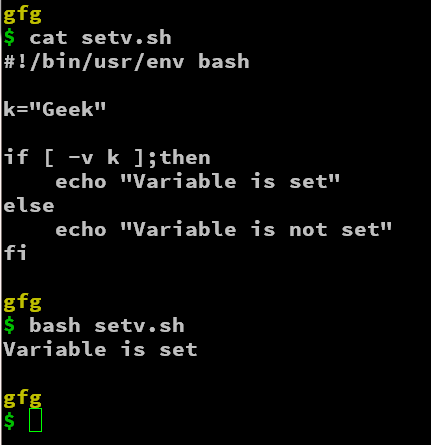As we can see in the above output the condition returns true if the variable is defined. Even if the variable might be an empty string, it will return true.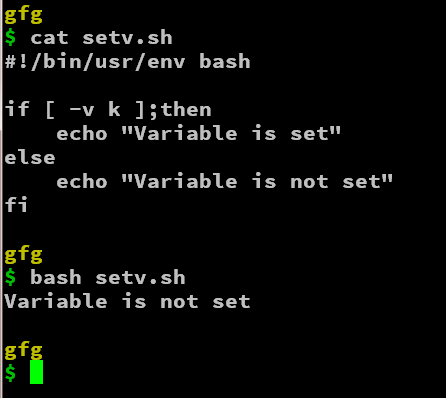Now, in this example, the variable k was not declared. Hence the option -v returns false and we evaluate the condition accordingly.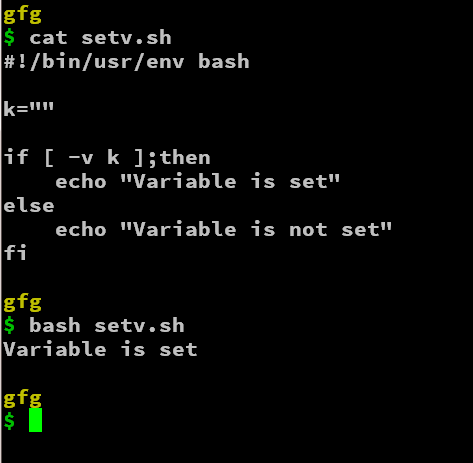In this last, example, we have declared the variable k as an empty string. And this time, as said, the -v option will return true if the variable was declared before, even if it was empty it will check if the variable was previously declared or not. Hence, we can conclude that the -v option returns true if the variable provided to it is declared irrespective of the value it contains.

## Using the “-z” option

The -z option allows us to check if the variable is of non-zero length or not. It returns true if the length of the value in the variable is zero (empty). Unlike, the -v option, it will return true if the string is empty. The syntax of this command is a little different and awkward than other options,

`[ -z "\${variable_name}" ]`

OR

`[[ -z \${variable_name} ]] `

Both of the syntax-es work a bit differently in certain situations like you don’t have to double-quote the variable if parsing in conditional statements. The choice is dependent on the user if portability in BASH is your priority, then the “[[” syntax works very well. Let’s look at the examples for cases when the variable is non-empty, not declared, and empty in a sample BASH script.

```#!/bin/usr/env bash

k="Geeks"

if [[ ! -z \${k} ]];then
echo "Variable is not empty"
else
echo "Variable is empty"
fi```

We have used “!” in front of -z so as to complement the condition, this is done to provide homogeneity in understanding different options and arguments.

Note: The -z options return true if the length of the value of the variable is zero, and the -v option returns true if the variable is declared earlier(i.e. it returns false for empty and non declared variables)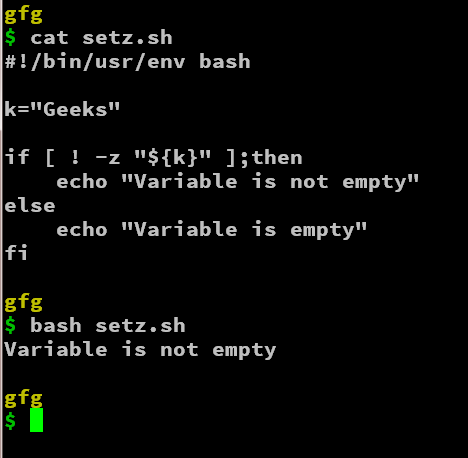As we can see, the -z option returned false i.e the variable value is not empty and hence we print the same. Since we have used the “!” operator ( not operator) we get the opposite of the evaluated expression.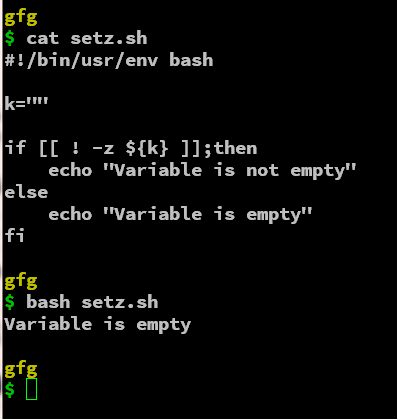As said, the -z option returned true i.e the variable’s value is empty. As in the conditional statement, the not operator reverses the result and we echo out that the variable is empty.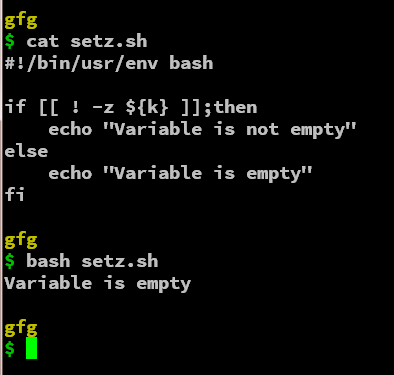In the above example, as the variable “k” is not defined or declared anywhere the -z option will return true as the variable itself doesn’t exist. Thus, we have seen the -z option returns true if the variable’s value is of length zero or is not defined

## Using the “-n” option

The -n option is kind of a combination of the -v and -z options, the -n option returns true if the value of the variable provided is non-empty.

• Unlike the -z option which returned false if the value of the variable is non-empty.
• Like the -z option, the -n option returns false if the value is declared but is empty.
• Unlike, the -v option, the -n option returns false if the variable is declared but has an empty value.
• Like the -v option, the -n option returns true if the value of the variable is non-empty.

Let us see the syntax of the -n option.

`[ -n "\${variable_name}" ]`

OR

`[[ -n \$variable_name ]] `

The syntax is not as strict as said, but it is recommended for any critical bugs in certain situations.

Now, we will see the cases like non-empty, not declared, and empty values of the variables passed to the -n option in the sample BASH script.

```#!/bin/usr/env bash

k="Geeks"

if [[ -n \$k ]];then
echo "Variable is set"
else
echo "Variable is not set"
fi```

The -n option will return true if the value of the variable is non-zero. If the value is empty or the variable is not declared, it will return false.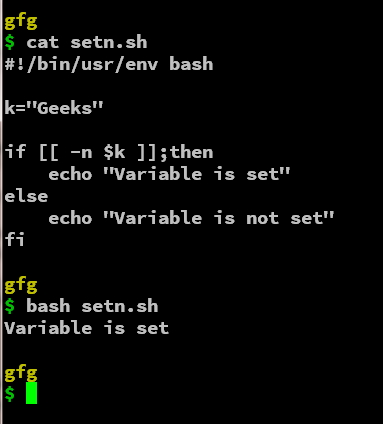As we can see that the condition returned true as the variable’s value(k) is non-empty.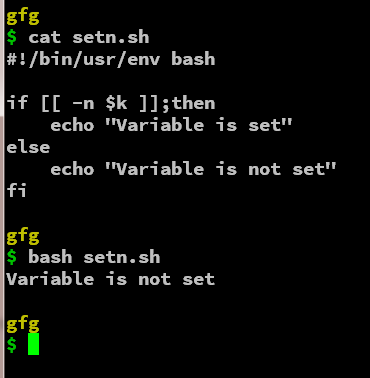In this example, as the variable (k) was not declared, the condition is evaluated as false and we echo out as the variable is not set.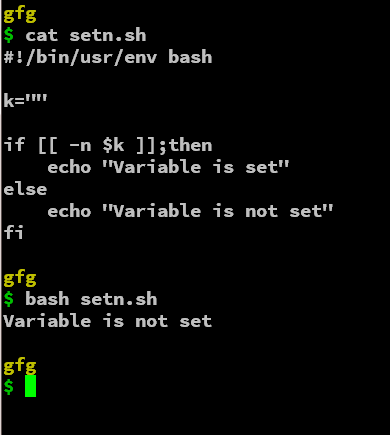In the above example, the variable(k) is empty and thus the condition using the -n option is evaluated to false and we echo out the context as per requirement.

From the above sets of examples, we were able to understand the different options like -z, -v, and -n to check if the variable is set/empty or not in a BASH script. The below table can be summarized for a clear understanding of the three options available.

## Modifiers for -z and -n options

We can have certain modifiers like +set and -unset to change the way the options evaluate the condition. We will look at them in short examples as below.

### +set modifier

We can set the modifier +set to the -z option to only return true if the variable is not declared and return false to set and empty values

`[[ -z \${variable_name+set} ]]`

We will apply the modifier in the example and evaluate the different result conditions generated because of the modifier attached to the -z option.

```#!/bin/usr/env bash

k=""

if [[ ! -z \${k+set} ]];then
echo "Variable is not empty"
else
echo "Variable is empty"
fi```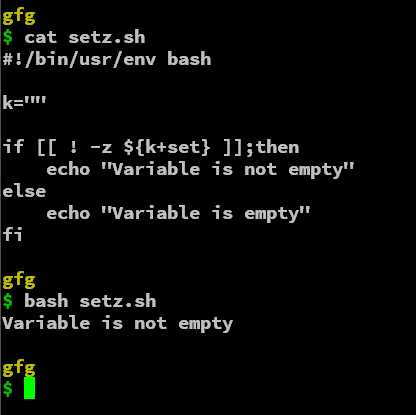We can see that the -z option along with the +set modifier returns false. As said earlier, we use the “!” operator to modify the condition and evaluate the results accordingly. Thus, the +set modifier only allows the set and empty values of the variable to be true and non-set values as false just like the -v option but in a reverse way

Now, the +set modifier can also be applied to the -n option. This modifier will allow the empty set variable to return true instead of false

`[[ -n \${variable_name+set} ]] `

We will apply the modifier in the example and evaluate the different result conditions generated because of the modifier attached to the -n option.

```#!/bin/usr/env bash

k=""

if [[ -n \${k+set} ]];then
echo "Variable is set"
else
echo "Variable is not set"
fi```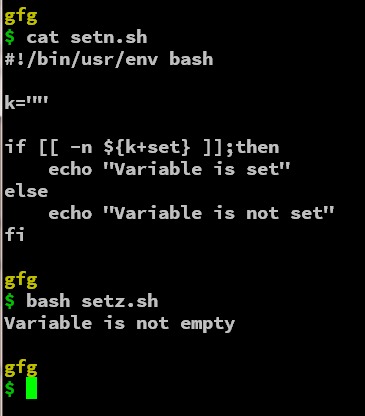The +set modifier is used with the -n option, this allows the empty set variable to evaluate the result as true. This modifies the -n option as the -v option which also accepts the empty string as true.

The other modifier is -unset, it allows the non-set values of variables to return true.  The syntax of the -unset modifier with the -z option is as given below:

`[[ -z \${variable_name-unset} ]]`

We will use the same example to demonstrate the usage of the -unset modifier for the -z option.

```#!/bin/usr/env bash

if [[ ! -z \${k-unset} ]];then
echo "Variable is not empty"
else
echo "Variable is empty"
fi```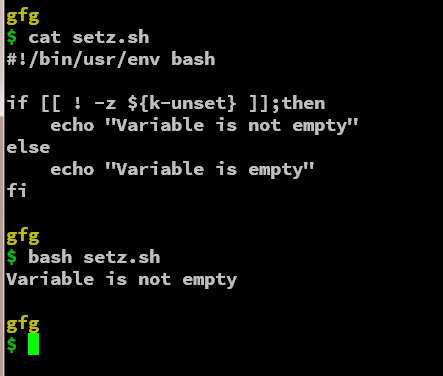The -unset modifier allows the -z option to accept the undeclared variable as false. This might not be very helpful but still is available if needed in certain situations.

We can even use the -unset modifier in -n option. The -unset modifier will allow the -n option to accept the not set or not declared variables to evaluate to true. The syntax is similar for the -n option with the -unset modifier.

`[[ -n \${variable_name-unset} ]]`

We will evaluate the modifier with the -n option with an example as given below:

```#!/bin/usr/env bash

if [[ -n \${k-unset} ]];then
echo "Variable is set"
else
echo "Variable is not set"
fi```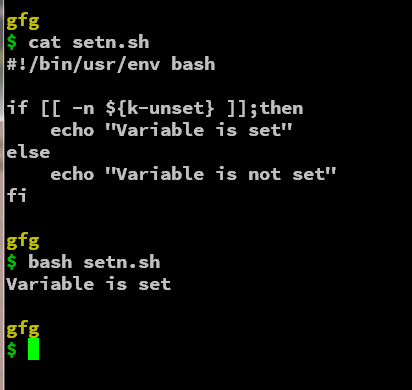As we can see, the -n option along with the -unset modifier allows the not set or not declared variable to return the condition as true. Thus this can be again an option to verify a condition provided with certain non-declared variable conditions and constraints.

Below is the modified chart to summarize the following options and modifiers.